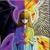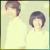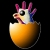## 我是不是要把C++所有的错误都犯一遍呢？

Timor君 发布于 2012/07/18 06:21

```class A
{
public:

virtual ~A(){}
};

class B : public A
{
private:
int m_b;
};

int main()
{
A *p = new B;
delete []p;

return 0;
}```

error: core dumped!

2

c++ 规定 delete [] 不履行多态。“ arrays are not treated polymorphically

delete [] p；这行会出错。

==============

§5.3.5/3 C++03 ：In the first alternative (delete object), if the static type of the operand is different from its dynamic type, the static type shall be a base class of the operand’s dynamic type and the static type shall have a virtual destructor or the behavior is undefined. In the second alternative (delete array) if the dynamic type of the object to be deleted differs from its static type, the behavior is undefined.

==============

==============

0

int main()
{
std::vector<B*> b(10); //10个元素
std::for_each(b.begin(), b.end(), [](B*& it) { it = new D; }); //循环new。比较讨厌

b->foo();  //不会出错

std::for_each(b.begin(), b.end(), [](B* it) { delete it; });     //循环delete 。比较讨厌。

return 0;
}

0delete看的应该是静态类型 而不是动态类型
0```#include <iostream>
using namespace std;
class Base
{
public:
Base():i(1){}
int get() {return i;}
private:
int i;
};

class Derived : public Base
{
public:
Derived():j(2){}

private:
int j;
};

int main()
{
Base b;
Derived d;
for(int i = 0; i < 5; i++)
{
b[i] = d[i];
cout << b[i].get() <<endl;
}

cout<<"-----------------------"<<endl;

Base *p = d;
for(i = 0; i < 5; i++)
cout << p[i].get() << endl;

return 0;
}```

R

0C++本身就是错误